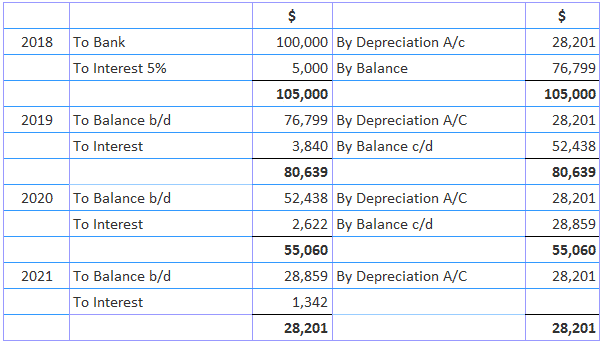# Annuity Method of Assets Depreciation### Written byTrue Tamplin, BSc, CEPF®

Updated on July 22, 2022

## Definition

In the annuity method of assets depreciation, it is supposed that the money invested in the purchase of the asset earns interest at a fixed rate.

This is debited to the asset account and, according to the annuity table, a certain fixed amount of depreciation is charged everywhere for the estimated useful life of the asset.

## Explanation

The amount of depreciation calculated by the annuity table at a given rate of interest includes the element of interest. It remains the same throughout the life of the asset, while interest taken forward will decrease in the coming years.

The method is followed where a large sum of money is required for the purchase of the asset and the life of the asset is definite (e.g., leasing). However, it is unsuitable for assets where additions to the asset are made during the course of the life of the asset off and on.

The method is based on a comprehensive interpretation of the term “cost of the asset.” An asset’s cost is defined as its purchase price plus the interest on the capital investment in the asset that would have been earned on the amount invested.

### Merits of Annuity Method of Assets Depreciation

1. Use of Interest: Unlike the traditional methods of providing depreciation, the annuity method considers interest on capital invested in the asset. 2. Exact and Suitable Method: It is regarded as the most exact and precise method from the point of view of calculations. 3. Scientific Method: The method is the most scientific because it takes interest into consideration.

### Demerits of the Annuity Method

1. Too Many Calculations: It depends on a number of complex calculations. 2. Not Suitable for Addition in Between: If the asset requires frequent additions and extensions, the calculations must be revised quite substantially after further complicating its application and operation. 3. Fixed Amount of Depreciation: Has the tendency to unequally charge to profit and loss account in respect of depreciation and repairs combined, which is because the amount of depreciation remains fixed over the period of the life of the asset.

### Application of the Annuity Method of Assets Depreciation

The method is well applicable to assets that require considerable investment, do not call for frequent additions, and do not need replacement (e.g., long-term leases). It is not suitable for plants and machinery that require frequent additions and replacement.

### Example

A lease was acquired for \$100,000 in 2018. Its working life is only 4 years. Depreciation under the annuity system at 5% p.a. interest is charged. \$28,201 is payable over 4 years @ 5% p.a., show the lease A/C.

### Solution

Depreciation = \$100,000 x 0.282012 = \$28,201## Annuity Method of Assets Depreciation FAQs